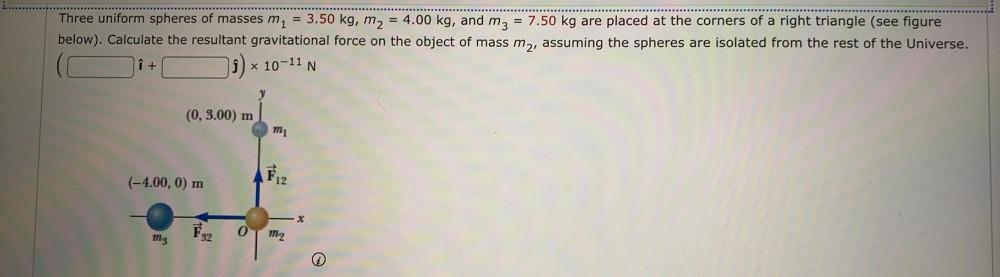Question:

# Three uniform spheres of masses mi = 3.50 kg, m2 = 4.00 kg, and m3 = 7.50 kg are placed at the corners of a right triangle (seeThree uniform spheres of masses mi = 3.50 kg, m2 = 4.00 kg, and m3 = 7.50 kg are placed at the corners of a right triangle (see figure below). Calculate the resultant gravitational force on the object of mass m2, assuming the spheres are isolated from the rest of the Universe. i + j) x 10-11 N (0, 3.00) m mi (-4.00, 0) m & 12 X o mg 32 m2